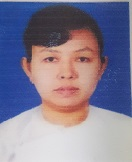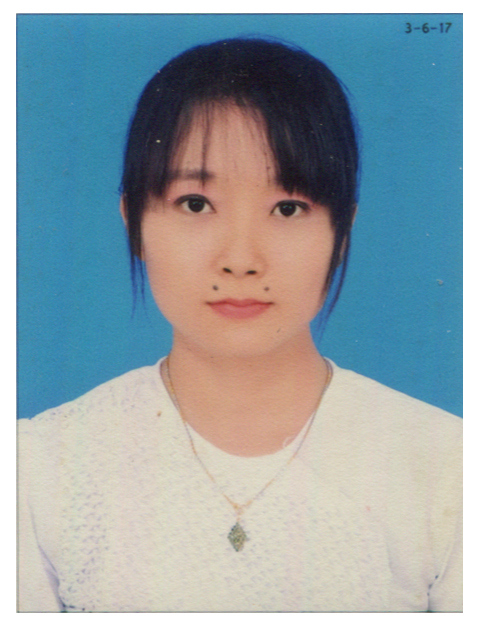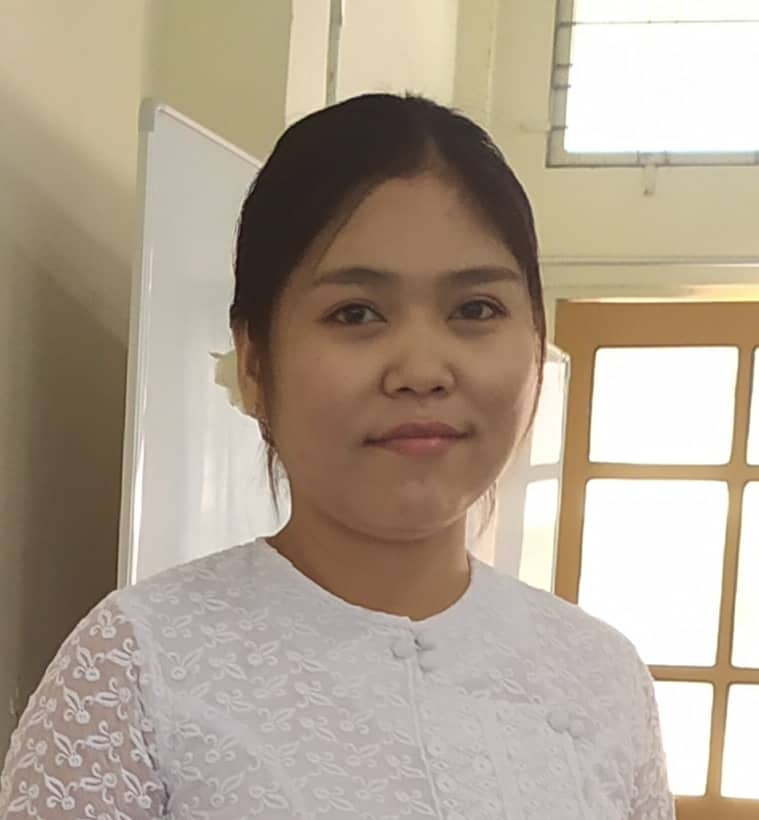UCSY[Faculty of Computing]  [Research Lab]

#### Numerical Analysis Lab

Numerical Analysis Laboratory implemented by Department of Computational Mathematics.

#### Objective

• To be able to implement the iteration process formula by using Numerical Analysis for the problem such as scientific problems, Information Technology problems ,management and Decision Solving Problems
• To research computerized solving system by using Numerical iteration process for implemented iteration process model practically
• To be able to use numerically solving tools for the iteration process model practically

#### Members of Numerical Analysis LabDr. May Aye Khine Professor and Head Numerical AnalysisDr. Khin Myo Myo Associate Professor Numerical AnalysisDaw Ni Ni Hla Associate Professor Numerical AnalysisDaw Kyi Kyi Win Lecturer Numerical AnalysisDaw Myint Myint Than Lecturer Numerical AnalysisDaw Thaw Thaw Nyein Lecturer Numerical AnalysisDaw ZuZan Ko Ko Assistant Lecturer Numerical AnalysisDaw Zin Mar Tun Assistant Lecturer Numerical AnalysisDaw Soe Yu Maw Assistant Lecturer Numerical AnalysisDaw May Myat Mon Staff officer Numerical AnalysisDaw Mi Khine Oo Assistant Lecturer Numerical AnalysisDaw Khin Swe Yin Assistant Lecturer Numerical AnalysisDaw Eaint Mon Win Assistant Lecturer Numerical Analysis
News
Computer Universities